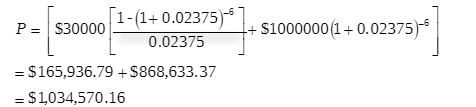# A funds manager forecasts that it will need to invest $1 million in approximately 90 days. The manager wishes to receive a return as close as possible to the medium-term interest rates currently avail... A funds manager forecasts that it will need to invest$1 million in approximately 90 days. The manager wishes to receive a return as close as possible to the medium-term interest rates currently available, but expects that rates will have fallen by the time the funds are available for investment.

(a) Outline what the manager would do today in the financial futures market in order to secure a return that is close to current medium-term market rates.

(b) Calculate the price of a three-year Treasury bond futures contract quoted at 95.25. A Commonwealth Treasury bond futures contract is based on a 6.00% per annum fixed interest bond with a face value of $1million and paying half-yearly coupons. (c) Outline and explain the factors that will determine how successful this strategy will be in securing an effective return that is close to today’s market rates. #### Homework Answers ReportAnswer #1 Answer a) In such condition , the fund managers may use different strategies to hedge the risk of interest rate fall . Some popular strategies are : Use of future contracts, fund manager investing in medium-term so can use a 3 years Commonwealth Treasury bond futures contracts. Answer b) Price of Future contract bond, P =i= interest rate per period expressed as a decimal =4.75% per annum / 2 = 2.375 = 0.02375 n = number of coupon periods = 3 years * 2 = 6 C = periodic coupon payments = 6% per annumm = 3% half yearly =$1million x 0.03 = $30 000 A = the face value =$1 000 000

putting the values in above formula,• The cost of the futures strategy should be collected from the client separately  as this will lower the net yield received.
• The hedging strategy may exposed risk , which impact the actual outcome of the strategy.
• An element of cross-commodity risk may be found , if used other commodity for hedging rather than treasury bill.